# Excel Find Function

This post will guide you how to use Excel FIND function with syntax and examples in Microsoft excel.

## Description

The Excel FIND function returns the position of the first text string (sub string) within another text string.

It can be used when you want to get the position of a sub string inside another text string. so it will return a number that indicates the starting position of sub string that you are searching in another text string.

The FIND function is a build-in function in Microsoft Excel and it is categorized as a Text Function.

Note: The FIND Function is case-sensitive.

The FIND function is available in Excel 2016, Excel 2013, Excel 2010, Excel 2007, Excel 2003, Excel xp, Excel 2000, Excel 2011 for Mac.

## Syntax

The syntax of the FIND function is as below:

`= FIND(find_text, within_text,[start_num])`

Where the FIND function arguments are:

• Find_text -This is a required argument. The text  or substring that you want to find. (The string in the Find_text argument can not contain any wildcard characters)
• within_text -This is a required argument. The text string that is to be searched
• start_num-This is an optional argument. It will specify the position in within text string where the search will start. If you omit start_num value, the search will start from the first character of the within_text string, in other words, it is set to be 1 by default.  So you can use the Excel Find function to look for the specified text starting from the specified position.

Note:

• The Find function will return the position of the first character of find_text in within_text argument.
• If there are several occurrences of the find_text within another text string, it only return the position of the first occurrence.
• If the find_text is an empty string, the position of the first character in the within_text is returned.
• If the find_text string is not found in within_text, it will return the Excel #VALUE! Error.
• If start_number value is not greater than 0, it will return the #VALUE! Error value.
• As the FIND function is case-sensitive. If you want to do a search that is case-insensitive , you can use the SEARCH Function.
• The FIND function does not support wildcard characters, so if you want to use wildcard characters to find string, you can use SEARCH function in excel.

## Excel Find Function Examples

The below example will show you how to use Excel FIND Text function to find the position of a sub string within text string.

#1 To get the position of sub string “how” in cell B1, just using formula:

` =FIND("how",B1,1).`

When you look for the text “how” in the Cell B1, it will return 6, which is the position of the first character of find_text “how” within the Cell B1.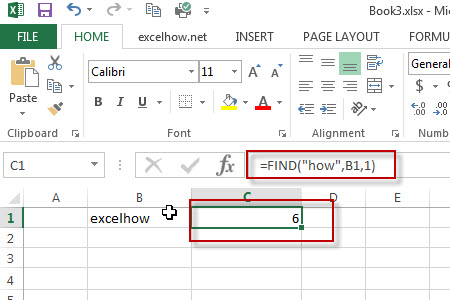#2 To get the position of sub string “how”in cell b1, starting with the third character, using formula:

`=FIND("how",B1,3)`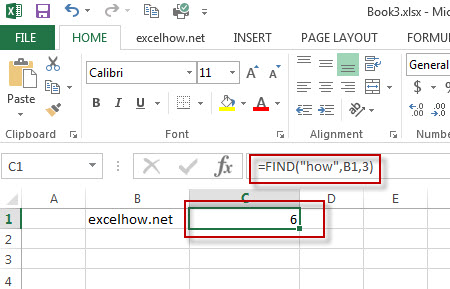In the above example, it specified the start_num value as “3“, so you will get the position of the first character “h” of  the searched string “how”  within the value in Cell B1 when the starting position is 3. it returns 6.

The Example 1The search will start from the third character of the value in Cell B1.

#3 Finding “excel” string  in Cell B1, using the following Find formula:

```=FIND("excel",B1,1)
=FIND("excel",B1,3)```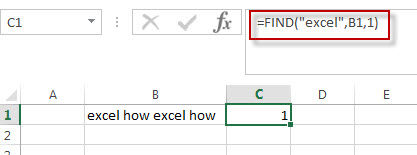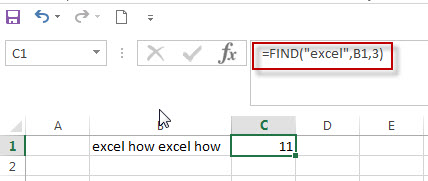when you set the starting position as 1, it will return the position of the first “excel” string in the value of Cell B1.

When you set the starting position as 3, it will return the position of the second “excel” string in the value of Cell B1. The search will start from the third character of the value in Cell B1.

#4 Searching an empty string in Cell B1, using the following Find formula:

`=FIND("",B1,1)`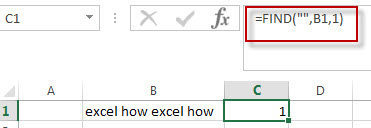In the above example, when you look for an empty string in Cell B1 with the starting position as 1, and it will return the position of the first character of the within_ text (the value of Cell B1).

#5 Searching “word” text in Cell B1, using the following Find formula:

`=FIND("word", B1,1)`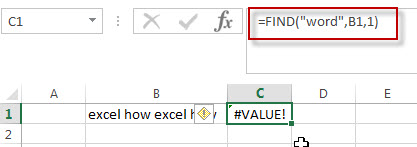In the above example, when you look for the text “word” in Cell B1 and the string position is 1, it will return a #VALUE! error, As the searched string “word” is not found in Cell B1.

#6 Searching for a text as “excel” in Cell B1 and the starting position is a negative number.

`=FIND("excel",B1,-1)`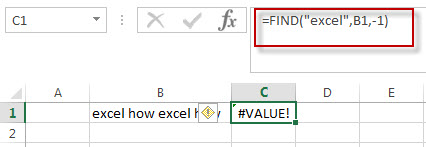If the starting position is not greater than 0, it will return a #VALUE! Error.

#7 Searching for a string as “EXCEL” in Cell B1, using the following formula:

`=FIND("EXCEL",B1,1)`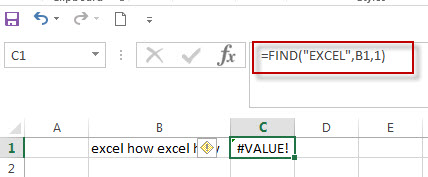As the FIND function in excel is case-sensitive, when you look for the string “EXCEL” in Cell B1, the searched string “EXCEL” is not found, the function will return a #VALUE! error.

Question 1:  the FIND function returns a #VALUE error when it do not find the searched text within another string.  I want to know if there is another excel function in combination with the find function to handle the error message to return the actual value, such as: -1 or throwing others exceptions via returning values, like: -1,0 or FALSE.

Answer: As mentioned above, the FIND function is case-sensitive, and it can be combined with the ISNUMBER function and IF function to create a new IF excel formula as follows:

`=IF(ISNUMBER( FIND("excel",B1)), FIND("excel",B1), "not found")`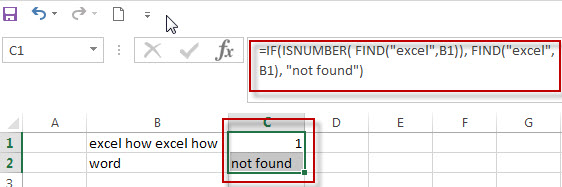The FIND function will locate the position of the searched text “excel” within Cell B1, and the ISNUMBER function will check the result returned by the FIND function if it is a number, if TRUE, then return TRUE, otherwise , returns FALSE. then the IF will check the Boolean value returned by ISNUMBER function, If TRUE, then returns the numeric position returned by the second FIND function, If FALSE, returns “not found” string.

If you want a case-insensitive match and you can use the SEARCH function instead of the FIND function in the above formula:

`=IF(ISNUMBER( SEARCH("excel",B1)), SEARCH("excel",B1), "not found")`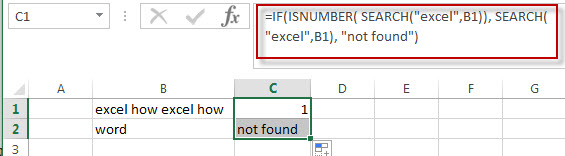Question 2: I want to extract a sub string from a string that separated by wildcard characters. I tried to use the below FIND formula to handle it. but it returned a #VALUE error message. Could you please help to check the below formula I used:

`=RIGHT(B1,FIND("~**",B1))`

Answer: you can use the following formula:

`=TRIM(RIGHT(B1,Len(B1) - FIND("**",B1)-1))`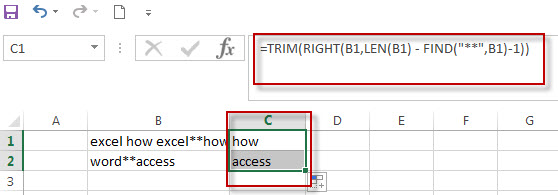The find function will return the position of the first wildcard (**) character within a string in Cell B1. you need subtract the numeric position returned by FIND function from the length of the string in Cell B1 to get the length of sub string to the right of the wildcard character(asterisk). then the RIGHT function extracts the rightmost characters based on the length of sub string.

or you can use another formula to achieve the same result as follows:

`=MID(B1,FIND("**",B1)+2,255)`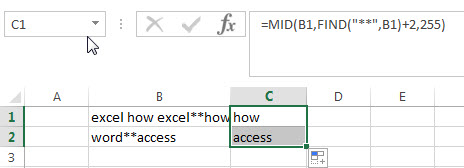Question 3: I am trying to extract the first word from another text string separated by space character. but it always return a #VALUE error message. I think it should be use the FIND function in combination with another function, such as: left function. but I still don’t know how to combine with those two functions to extract the first word.

Answer: right, you can create a excel formula that uses the FIND function and LEFT function. and you can use the below formula:

`=IFERROR(LEFT(B1, FIND(" ",B1)-1),B1)`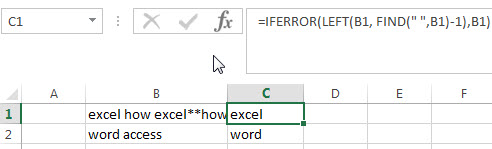The find function returns the position of the first space character in the text string in Cell B1. the Formula “=FIND(” “,B1)-1 returns the numbers of the first word as the second arguments of the LEFT function.

If the text string in Cell B1 just only contains one word, then the find function will return #VALUE error. to handle this error, you need to use the IFERROR function, if the find function is not find the space character, then return the text string in B1. in other words, it just contains only one word in Cell B1.

Question 4: I am trying to extract an email address from a text string in Cell B1. how to write a excel formula to achieve the result.

Answer: you can use a combination of the TRIM function, the LEFT function, the SUBSTITUTE function, the MID function, the MAX function and the REPT function to create a complex excel formula to extract email address from a string in Cell B1.

`=TRIM(RIGHT(SUBSTITUTE(LEFT(B1,FIND (" ",B1&" ",FIND("@",B1))-1)," ", REPT(" ",LEN(B1))),LEN(B1)))`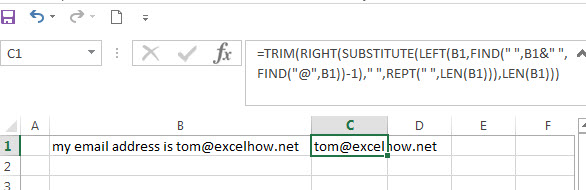`=TRIM( MID( SUBSTITUTE(B1, " ", REPT(" ",255)), MAX(1, FIND( "@", SUBSTITUTE(B1, " ", REPT(" ",255))) -50),255))`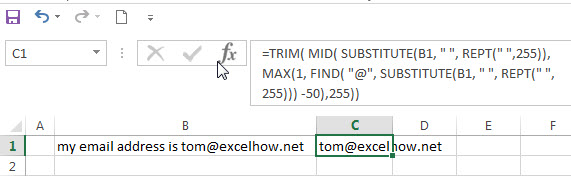Question 5: I am trying to get the first name from a full name separated by a comma character. how to use a excel formula to extract the first name from a name as: “Last name, First name” format.

Answer: you can use a combination with the RIGHT function, the LEN function and the FIND function to create a excel formula to get the first name from a name. just using the following formula:

`=RIGHT(B1,LEN(B1) - FIND(", ",B1)-1)`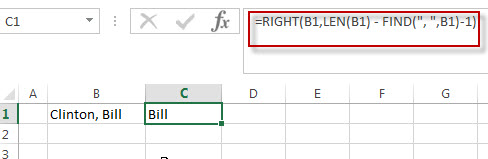### Related Functions

• Excel SEARCH function
The Excel SEARCH function returns the number of the starting location of a substring in a text string.The syntax of the SEARCH function is as below:= SEARCH  (find_text, within_text,[start_num])…
• Excel Find function
The Excel FIND function returns the position of the first text string (substring) from the first character of the second text string.The FIND function is a build-in function in Microsoft Excel and it is categorized as a Text Function.The syntax of the FIND function is as below:= FIND  (find_text, within_text,[start_num])…
• Excel ISERROR function
The Excel ISERROR function returns TRUE if the value is any error value except #N/A. The ISERROR function is a build-in function in Microsoft Excel and it is categorized as an Information Function. The syntax of the ISERROR function is as below: = ISERROR (value) …
• Excel ISNUMBER function
The Excel ISNUMBER function returns TRUE if the value in a cell is a numeric value, otherwise it will return FALSE.The syntax of the ISNUMBER function is as below:= ISNUMBER (value)…
• Excel RIGHT function
The Excel RIGHT function returns a sub string (a specified number of the characters) from a text string, starting from the rightmost character.The syntax of the RIGHT function is as below:= RIGHT (text,[num_chars])…
• Excel LEN function
The Excel LEN function returns the length of a text string (the number of characters in a text string).The LEN function is a build-in function in Microsoft Excel and it is categorized as a Text Function.The syntax of the LEN function is as below:= LEN(text)…
• Excel MID function
The Excel MID function returns a sub string from a text string at the position that you specify. The MID function is a build-in function in Microsoft Excel and it is categorized as a Text Function. The syntax of the MID function is as below: = MID (text, start_num, num_chars) …
• Excel SUBSTITUTE function
The Excel SUBSTITUTE function replaces a new text string for an old text string in a text string.The syntax of the SUBSTITUTE function is as below:= SUBSTITUTE  (text, old_text, new_text,[instance_num])…
• Excel LEFT function
The Excel LEFT function returns a sub string (a specified number of the characters) from a text string, starting from the leftmost character.The LEFT function is a build-in function in Microsoft Excel and it is categorized as a Text Function.The syntax of the LEFT function is as below:= LEFT(text,[num_chars])…
• Excel REPT function
The Excel REPT function repeats a text string a specified number of times.The REPT function is a build-in function in Microsoft Excel and it is categorized as a Text Function.The syntax of the REPT function is as below:= REPT  (text, number_times)…

### More FIND Formula Examples in Excel

• Split Text String by Specified Character
you should use the Left function in combination with FIND function to split the text string in excel. And we can refer to the below formula that will find the position of “-“in Cell B1 and extract all the characters to the left of the dash character “-“.=LEFT(B1,FIND(“-“,B1,1)-1).…
• Split Text String by Line Break
When you want to split text string by line break in excel, you should use a combination of the LEFT, RIGHT, CHAR, LEN and FIND functions. The CHAR (10) function will return the line break character, the FIND function will use the returned value of the CHAR function as the first argument to locate the position of the line break character within the cell B1.…
• Split Text and Numbers in Excel
If you want to split text and numbers, you can run the following excel formula that use the MIN function, FIND function and the LEN function within the LEFT function in excel. .…
• Get the Current Worksheet Name only
If you want to get the current worksheet name only in excel, you can use a combination of the MID function, the CELL function and FIND function.…
• Get full File Name (workbook and worksheet) and Path
In excel, you can get the current workbook name and it is absolute path using the CELL function.  Just refer to the following formula:=CELL(“filename”,B1).…
• Get the Current Workbook Name
If you want to get the name of the current workbook only, you can use a combination of the MID function, the CELL function and the FIND Function…
• Get Path and Workbook name only
If you want to get the workbook name and its path only without worksheet name, you can use a combination of the SUBSTITUTE function, the LEFT function, the CELL function and the FIND function….
• Get First Name From Full Name
If you want to get the first name from a full name list in Column B, you can use the FIND function within LEFT function in excel. The generic formula is as follows:=LEFT(B1,FIND(” “,B1)-1).…
• Get Last Name From Full Name
To extract the last name within a Full name in excel. You need to use a combination of the RIGHT function, the LEN function, the FIND function and the SUBSTITUTE function to create a complex formula in excel..…
• The different between FIND and SEARCH functions
There are two big differences between FIND and SEARCH functions in excel.The FIND function is case-sensitive. And the SEARCH function is case-insensitive……
• Data Validation for Specified Text only
If you want to check if the values that contain a specified text string in one cell, you can use a combination of the FIND function and ISNUMBER function as a formula in the Data Validation….…
• Count Cells That Contain Specific Text
This post will discuss that how to count the number of cells that contain specific text or certain text in a specified cells of range in Excel. How to get the total number of cells that contain certain text.……
• Split Text and Numbers
If you want to split text and numbers from one cell into two different cells, you can use a formula based on the FIND function, the LEFT function or the RIGHT function and the MIN function. .…
• Quickly Get Sheet Name
If you want to quickly get current worksheet name only, then inert it into one cell. You can use a formula based on the MID function in combination with the FIND function and the Cell function……